# Modus ponens and Modus tollens

Modus Ponens and Modus Tollens are forms of valid inferences. By Modus Ponens, from a conditional statement and its antecedent, the consequent of the conditional statement is inferred: e.g. from “If John loves Mary, Mary is happy” and “John loves Mary,” “Mary is happy” is inferred. By Modus Tollens, from a conditional statement and the negation of its consequent, the negation of the antecedent of the conditional statement is inferred: e.g. from “If today is Monday, then tomorrow is Tuesday” and “Tomorrow is not Tuesday,” “Today is not Monday” is inferred. The validity of these inferences is widely recognized and they are incorporated into many logical systems.

## Modus Ponens

Modus Ponens (Latin: mode that affirms; often abbreviated as MP) is a form of valid inference. An instance of MP inferences involves two premises: One is a conditional statement, i.e. a statement of the form If A, then B; the other is the affirmation of the antecedent of the conditional statement, i.e. A in the conditional statement If A, then B. From these such pairs of premises, MP allows us to infer the consequent of the conditional statement, i.e. B in If A then B. The validity of such inferences is intuitively clear, since B must be true if the statements, If A, then B and A are both true.

Here is an example of an MP inference:

If Jack is innocent, he has an alibi.
Jack is innocent.
Therefore, Jack has an alibi.

The first two statements are the premises and the third statement is the conclusion. If the first and second are true, we are forced to accept the third.

One thing that may be mentioned here is that, in general, the validity of an inference does not guarantee the truth of the statements in the inference. The validity only assures us the truth of the conclusion assuming that the premises are true. Thus, for instance, it may be the case that not every innocent suspect has an alibi and that the first statement of the above example of MP inferences is in fact false. However, this does not affect the validity of the inference, since the conclusion must be true when we assume the two premises are true regardless of whether the two premises are in fact true.

The concept that involves the truth of the premises of inferences is soundness. An inference is sound if it is valid and all the premises are true; otherwise, the inference is unsound. Thus, an argument can be unsound even if it is valid, since valid arguments can have false premises.

Modus Ponens is referred to also as Affirming the Antecedent and Law of Detachment.

## Modus Tollens

Modus Tollens (Latin for "mode that denies" abbreviated as MT) is another form of valid inference. As in the case of MP, an instance of MT inferences involves two premises. One is again a conditional statement If A then B, while the other, unlike MP, is the negation of the consequent, i.e. a statement of the form not B. From such pairs of premises, MT allows us to infer the negation of the antecedent of the conditional statement, i.e. not A. To see the validity of such inferences, assume toward contradiction that A is true given the two premises, If A then B and not B are true. Then, by applying MP to A and If A then B, we can derive B. This is contradictory and thus A is false, i.e. not A.

Here is an example of an MT inference

If Jack is innocent, he has an alibi.
Jack does not have an alibi.
Therefore, Jack is not innocent.

MT is often referred to also as Denying the Consequent. (Note that there are kinds of inferences that are similarly-named but invalid, such as Affirming the Consequent or Denying the Antecedent.)

## Formal Representations

MP and MT are widely recognized as valid and, in fact, there are various kinds of logic that validate both of them. Formal representations of these forms of inferences are given by using the language of propositional logic: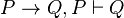$P \rightarrow Q, P \vdash Q$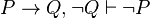$P \rightarrow Q, \lnot Q \vdash \lnot P$

(where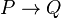$P \rightarrow Q$ represents the conditional statement If P then Q,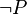$\lnot P$, the negation of P; and$\vdash$ means that, from the statements on the left side of it, the right side can be derived.) Particularly, MP is so fundamental that it is often taken as a basic inferential rule of logical systems (while MT is usually a rule that can be derived by using basic ones in most of the logical systems). Here, we present several different formal representations of MP.

Natural Deduction

P → Q    P
Q

Sequent Calculus (MP is usually called Cut in sequent calculus.)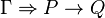$\Gamma \Rightarrow P \rightarrow Q$$\Delta \Rightarrow P$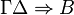$\Gamma \Delta \Rightarrow B$